HomeCompositio Mathematica

# Composite quasianalytic functions

Published online by Cambridge University Press:  17 August 2018

Corresponding

## Abstract

We prove two main results on Denjoy–Carleman classes: (1) a composite function theorem which asserts that a function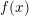$f(x)$ in a quasianalytic Denjoy–Carleman class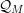${\mathcal{Q}}_{M}$ , which is formally composite with a generically submersive mapping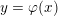$y=\unicode[STIX]{x1D711}(x)$ of class${\mathcal{Q}}_{M}$ , at a single given point in the source (or in the target) of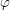$\unicode[STIX]{x1D711}$ can be written locally as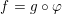$f=g\circ \unicode[STIX]{x1D711}$ , where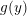$g(y)$ belongs to a shifted Denjoy–Carleman class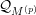${\mathcal{Q}}_{M^{(p)}}$ ; (2) a statement on a similar loss of regularity for functions definable in the$o$ -minimal structure given by expansion of the real field by restricted functions of quasianalytic class${\mathcal{Q}}_{M}$ . Both results depend on an estimate for the regularity of a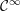${\mathcal{C}}^{\infty }$ solution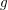$g$ of the equation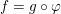$f=g\circ \unicode[STIX]{x1D711}$ , with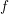$f$ and$\unicode[STIX]{x1D711}$ as above. The composite function result depends also on a quasianalytic continuation theorem, which shows that the formal assumption at a given point in (1) propagates to a formal composition condition at every point in a neighbourhood.

## MSC classification

Type
Research Article
Information
Compositio Mathematica , September 2018 , pp. 1960 - 1973

## Access options

Get access to the full version of this content by using one of the access options below.

## References

Belotto da Silva, A., Biborski, I. and Bierstone, E., Solutions of quasianalytic equations , Selecta Math. (N.S.) 23 (2017), 25232552.CrossRefGoogle Scholar
Bierstone, E. and Milman, P. D., Semianalytic and subanalytic sets , Publ. Math. Inst. Hautes Études Sci. 67 (1988), 542.CrossRefGoogle Scholar
Bierstone, E. and Milman, P. D., Canonical desingularization in characteristic zero by blowing up the maximum strata of a local invariant , Invent. Math. 128 (1997), 207302.CrossRefGoogle Scholar
Bierstone, E. and Milman, P. D., Resolution of singularities in Denjoy–Carleman classes , Selecta Math. (N.S.) 10 (2004), 128.CrossRefGoogle Scholar
Bierstone, E., Milman, P. D. and Valette, G., Arc-quasianalytic functions , Proc. Amer. Math. Soc. 143 (2015), 39153925.CrossRefGoogle Scholar
Borel, E., Sur la généralisation du prolongement analytique , C. R. Math. Acad. Sci. Paris 130 (1900), 11151118.Google Scholar
Carleman, T., Les fonctions quasi-analytiques, Collection Borel (Gauthier-Villars, Paris, 1926).Google Scholar
Chaumat, J. and Chollet, A., Sur la division et la composition dans des classes ultradifférentiables , Studia Math. 136 (1999), 4970.Google Scholar
Denjoy, A., Sur les fonctions quasi-analytiques de variable reélle , C. R. Math. Acad. Sci. Paris 173 (1921), 13291331.Google Scholar
Glaeser, G., Fonctions composées différentiables , Ann. of Math. (2) 77 (1963), 193209.CrossRefGoogle Scholar
Hadamard, J., Lectures on Cauchy’s problem in linear partial differential equations (Yale University Press, New Haven, 1923).Google Scholar
Hörmander, L., The analysis of linear partial differential operators I (Springer, Berlin–Heidelberg–New York, 1983).Google Scholar
Jaffe, E., Pathological phenomena in Denjoy–Carleman classes , Canad. J. Math. 68 (2016), 88108.CrossRefGoogle Scholar
Komatsu, H., The implicit function theorem for ultradifferentiable mappings , Proc. Japan Acad. Ser. A Math. Sci. 55 (1979), 6972.CrossRefGoogle Scholar
Malgrange, B., Frobenius avec singularités, 2. Le cas général , Invent. Math. 39 (1977), 6789.CrossRefGoogle Scholar
Mandelbrojt, S., Séries Adhérentes, Régularisation des Suites, Applications, Collection Borel (Gauthiers-Villars, Paris, 1952).Google Scholar
Miller, C., Infinite differentiability in polynomially bounded o-minimal structures , Proc. Amer. Math. Soc. 123 (1995), 25512555.CrossRefGoogle Scholar
Nowak, K. J., A note on Bierstone-Milman-Pawłucki’s paper ‘Composite differentiable functions’ , Ann. Polon. Math. 102 (2011), 293299.CrossRefGoogle Scholar
Nowak, K. J., On division of quasianalytic function germs , Int. J. Math. 13 (2013), 15.Google Scholar
Nowak, K. J., Quantifier elimination in quasianalytic structures via non-standard analysis , Ann. Polon. Math. 114 (2015), 235267.CrossRefGoogle Scholar
Rolin, J.-P., Speissegger, P. and Wilkie, A. J., Quasianalytic Denjoy–Carleman classes and o-minimality , J. Amer. Math. Soc. 16 (2003), 751777.CrossRefGoogle Scholar
Roumieu, C., Ultradistributions définies sur ℝ n et sur certaines classes de variétés différentiables , J. Anal. Math. 10 (1962–63), 153192.CrossRefGoogle Scholar
Thilliez, V., On quasianalytic local rings , Expo. Math. 26 (2008), 123.CrossRefGoogle Scholar

### Full text views

Full text views reflects PDF downloads, PDFs sent to Google Drive, Dropbox and Kindle and HTML full text views.

Total number of HTML views: 5
Total number of PDF views: 64 *
View data table for this chart

* Views captured on Cambridge Core between 17th August 2018 - 18th January 2021. This data will be updated every 24 hours.

Hostname: page-component-77fc7d77f9-94bw7 Total loading time: 0.31 Render date: 2021-01-18T21:36:52.345Z Query parameters: { "hasAccess": "0", "openAccess": "0", "isLogged": "0", "lang": "en" } Feature Flags last update: Mon Jan 18 2021 20:54:33 GMT+0000 (Coordinated Universal Time) Feature Flags: { "metrics": true, "metricsAbstractViews": false, "peerReview": true, "crossMark": true, "comments": true, "relatedCommentaries": true, "subject": true, "clr": true, "languageSwitch": true, "figures": false, "newCiteModal": false, "shouldUseShareProductTool": true, "shouldUseHypothesis": true, "isUnsiloEnabled": true }

# Send article to Kindle

Note you can select to send to either the @free.kindle.com or @kindle.com variations. ‘@free.kindle.com’ emails are free but can only be sent to your device when it is connected to wi-fi. ‘@kindle.com’ emails can be delivered even when you are not connected to wi-fi, but note that service fees apply.

Find out more about the Kindle Personal Document Service.

Composite quasianalytic functions
Available formats
×

# Send article to Dropbox

To send this article to your Dropbox account, please select one or more formats and confirm that you agree to abide by our usage policies. If this is the first time you use this feature, you will be asked to authorise Cambridge Core to connect with your <service> account. Find out more about sending content to Dropbox.

Composite quasianalytic functions
Available formats
×

# Send article to Google Drive

To send this article to your Google Drive account, please select one or more formats and confirm that you agree to abide by our usage policies. If this is the first time you use this feature, you will be asked to authorise Cambridge Core to connect with your <service> account. Find out more about sending content to Google Drive.

Composite quasianalytic functions
Available formats
×
×